Poisson Regression

The most widely used model for count data analysis is Poisson regression. This assumes that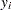, given the vector of covariates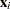, is independently Poisson-distributed with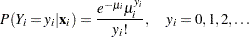and the mean parameter (that is, the mean number of events per period) is given by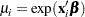whereis a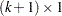parameter vector. (The intercept is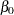; the coefficients for theregressors are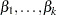.) Taking the exponential of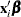ensures that the mean parameter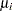is nonnegative. It can be shown that the conditional mean is given by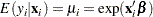The name log-linear model is also used for the Poisson regression model since the logarithm of the conditional mean is linear in the parameters: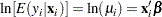Note that the conditional variance of the count random variable is equal to the conditional mean in the Poisson regression model: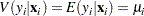The equality of the conditional mean and variance ofis known as equidispersion.

The marginal effect of a regressor is given by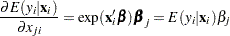Thus, a one-unit change in theth regressor leads to a proportional change in the conditional mean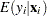of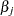.

The standard estimator for the Poisson model is the maximum likelihood estimator (MLE). Since the observations are independent, the log-likelihood function is written as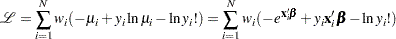where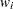is defined as follows:

1

if neither the WEIGHT nor the FREQ statement is used.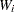whereare the nonnormalized values of the variable specified in the WEIGHT statement in which the NONORMALIZE option is specified.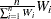whereare the nonnormalized values of the variable specified in the WEIGHT statement.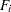whereare the values of the variable specified in the FREQ statement.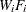if both the WEIGHT statement, without the NONORMALIZE option, and the FREQ statement are specified.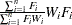if both the FREQ and the WEIGHT statements are specified.

The gradient and the Hessian are, respectively,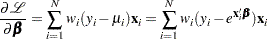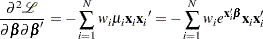The Poisson model has been criticized for its restrictive property that the conditional variance equals the conditional mean. Real-life data are often characterized by overdispersion (that is, the variance exceeds the mean). Allowing for overdispersion can improve model predictions since the Poisson restriction of equal mean and variance results in the underprediction of zeros when overdispersion exists. The most commonly used model that accounts for overdispersion is the negative binomial model.

Note: This procedure is experimental.